# Unpivot In Google Sheets With Formulas, Or How To Turn Wide Data Into Tall Data

Unpivot in Google Sheets is a method to turn “wide” tables into “tall” tables, which are more convenient for analysis.

Suppose we have a wide table like this: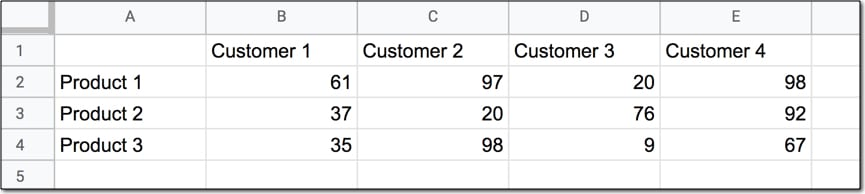We want to transform that data — unpivot it — into the tall format that is the way databases store data: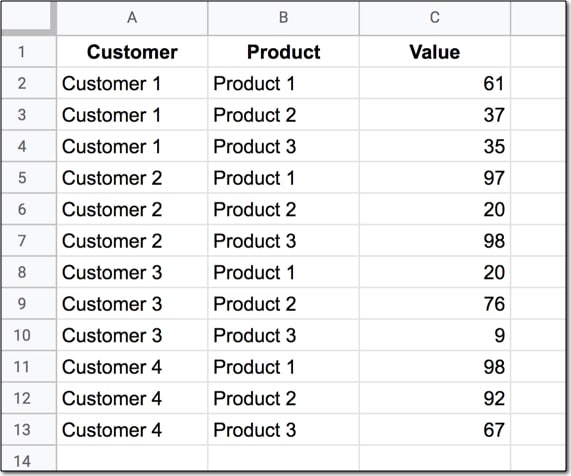But how do we unpivot our data like that?

It turns out it’s quite hard.

Much harder than going the other direction, pivoting tall data into wide data tables.

This article looks at how to do it using formulas, which is challenging and obtuse.

The formulas are complex and difficult to read so it’s hard to recommend this method in a production setting.

But it’s a fascinating look at advanced formulas in Google Sheets and I’m certain you’ll learn something new along the way.

If you need to do this in a production setting, then you might want to consider using the Apps Script code or example sheet from the first answer of this Stack Overflow post.

But if you’re ready for some complex formulas, let’s dive in…

## Unpivot in Google Sheets – Solution 1

We’ll use the wide dataset shown in the first image at the top of this post, in Sheet1 of our Google Sheet.

Remember, what we’re trying to do is transform the wide data table into the tall data table. The output of our formulas should look like the second image in this post.

In other words, we need to create 16 rows to account for the different pairings of Customer and Product, e.g. Customer 1 + Product 1, Customer 1 + Product 2, etc. all the way up to Customer 4 + Product 4.

Of course, we’ll employ the Onion Method to understand these formulas.

### Template

Feel free to make your own copy (File > Make a copy…).

(If you can’t open the file, it’s likely because your G Suite account prohibits opening files from external sources. Talk to your G Suite administrator or try opening the file in an incognito browser.)

### Customers Column

To start, create a second Sheet and add a simple header row in row 1, with “Customer”, “Product” and “Value” in cells A1, B1 and C1 respectively.

Let’s create an array formula to populate the customers column. In cell A2, enter this:

`=COUNTA(Sheet1!\$1:\$1)`

This formula gives the count of the number of columns — 4 — in our wide dataset (assuming cell A1 in our original dataset is empty, per the first image of this post).

QUICK NOTE: when copy-pasting these formulas into your own Google Sheets, paste them directly into the formula bar to avoid any issues.

Similarly, this next formula would give the count of the number of rows — 3 — in our wide dataset (again assuming cell A1 in our original dataset is empty, per the first image of this post).

`=COUNTA(Sheet1!\$A:\$A)`

Multiplying these two together gives us the number of values in our table — 12 — which corresponds to the number of rows we’ll need in our new tall data table:

`=COUNTA(Sheet1!\$A:\$A)*COUNTA(Sheet1!\$1:\$1)`

So let’s create those 12 rows!

Wrap this with the SEQUENCE function, starting from 1:

`=SEQUENCE(COUNTA(Sheet1!\$A:\$A)*COUNTA(Sheet1!\$1:\$1),1)`

Now divide that by the count of rows:

`=SEQUENCE(COUNTA(Sheet1!\$A:\$A)*COUNTA(Sheet1!\$1:\$1),1)/COUNTA(Sheet1!\$A:\$A)`

Hmm, it gives an answer of 0.3333333 but we’ve lost our 12 rows…

…so turn it into an Array Formula silly!

`=ArrayFormula(SEQUENCE(COUNTA(Sheet1!\$A:\$A)*COUNTA(Sheet1!\$1:\$1),1)/COUNTA(Sheet1!\$A:\$A))`

Ah, that’s better. “But how does it help us?” I hear you ask.

Let’s round all those decimals up to the nearest integer, like so:

`=ArrayFormula(ROUNDUP(SEQUENCE(COUNTA(Sheet1!\$A:\$A)*COUNTA(Sheet1!\$1:\$1),1)/COUNTA(Sheet1!\$A:\$A)))`

Nice!

We now have the column vector `1,1,1,2,2,2,3,3,3,4,4,4` with repeating positions, which is exactly what we needed.

For the moment, leave this formula alone and let’s move to cell B2 to construct the next piece. We want to create a table of the column headings that we can “lookup” with those repeating positions. Don’t worry, it’ll make more sense in a moment!

`=ArrayFormula(COLUMN(Sheet1!\$1:\$1))`

And try this formula in B3:

`=ArrayFormula(Sheet1!\$1:\$1)`

Can you see what we’re doing yet?

Let’s combine these in cell B2 as follows:

`=ArrayFormula({COLUMN(Sheet1!\$1:\$1);Sheet1!\$1:\$1})`

and delete the formula in cell B3.

The output should look the same, but it’s created with a single formula.

Now we can use the HLOOKUP function to lookup those positions into this data array we’ve created.

Change our formula in cell A2 to:

`=ArrayFormula(HLOOKUP(ROUNDUP(SEQUENCE(COUNTA(Sheet1!\$A:\$A)*COUNTA(Sheet1!\$1:\$1),1)/COUNTA(Sheet1!\$A:\$A)),{COLUMN(Sheet1!\$1:\$1);Sheet1!\$1:\$1},2))`

It’s nearly right, but the answer is offset slightly. Hmm.

Ah ok, it’s that blank cell in A1 of the original data that we didn’t account for. Our repeating positions really start from 2. It’s a simple fix to just add 1 to them.

`=ArrayFormula(HLOOKUP(ROUNDUP(SEQUENCE(COUNTA(Sheet1!\$A:\$A)*COUNTA(Sheet1!\$1:\$1),1)/COUNTA(Sheet1!\$A:\$A))+1,{COLUMN(Sheet1!\$1:\$1);Sheet1!\$1:\$1},2))`

That’s the customers populated in column 1.

What about the products in column 2?

### Products Column

Well, it’s an almost identical formula, so I’ll just share it here and leave it to the reader to use the Onion Method to build it in steps.

Actually, no I won’t, that’s just me being lazy. Let’s walk through it together.

It’s a similar idea, but it looks a little different because we do a vertical lookup.

So, if you haven’t already, clear out cells B2 and B3.

`=SEQUENCE(COUNTA(Sheet1!\$A:\$A)*COUNTA(Sheet1!\$1:\$1),1)`

This time we want a sequence that looks like 1,2,3,1,2,3,1,2,3 etc. i.e. repeating. This calls for the MOD squad, I mean MOD function.

`=MOD(SEQUENCE(COUNTA(Sheet1!\$A:\$A)*COUNTA(Sheet1!\$1:\$1),1),COUNTA(Sheet1!\$A:\$A))`

Oops, make it an Array Formula:

`=ArrayFormula(MOD(SEQUENCE(COUNTA(Sheet1!\$A:\$A)*COUNTA(Sheet1!\$1:\$1),1),COUNTA(Sheet1!\$A:\$A)))`

Ah, that’s better. But it gives 1,2,0,1,2,0 etc. so it’s not quite right. Fix the ordering by subtracting 1 from the dividend of the MOD function:

`=ArrayFormula(MOD(SEQUENCE(COUNTA(Sheet1!\$A:\$A)*COUNTA(Sheet1!\$1:\$1),1)-1,COUNTA(Sheet1!\$A:\$A)))`

Now we have 0,1,2,0,1,2 etc.

Add 2 to this to get the repeating positions we want 2,3,4,2,3,4 (again, we start from 2 to account for the blank cell in A1 of our original dataset).

`=ArrayFormula(MOD(SEQUENCE(COUNTA(Sheet1!\$A:\$A)*COUNTA(Sheet1!\$1:\$1),1)-1,COUNTA(Sheet1!\$A:\$A))+2)`

Leave this formula sitting pretty for a moment, and begin a new one in cell C2. Build an array for the vertical lookup with this formula (feel free to build in steps, I’m jumping straight to the array version):

`=ArrayFormula({ROW(Sheet1!\$A:\$A),Sheet1!\$A:\$A})`

Now we can combine this into the formula in cell B2, using a VLOOKUP:

`=ArrayFormula(VLOOKUP(MOD(SEQUENCE(COUNTA(Sheet1!\$A:\$A)*COUNTA(Sheet1!\$1:\$1),1)-1,COUNTA(Sheet1!\$A:\$A))+2,{ROW(Sheet1!\$A:\$A),Sheet1!\$A:\$A},2))`

Woohoo!

There’s our products in repeating order and paired correctly with the customer column.

That leaves the values associated with each pair.

### Values Column

Thankfully this is much simpler, using a standard INDEX / MATCH / MATCH construction to look up each pair.

The row offset in the INDEX function is found by matching the product with the product categories in column A of our original data, i.e.

`=MATCH(B2,Sheet1!\$A:\$A,0)`

The column offset is found by matching the customers, i.e.

`=MATCH(A2,Sheet1!\$1:\$1,0)`

Plug these both into the INDEX function:

`=INDEX(Sheet1!\$1:\$1000,MATCH(B2,Sheet1!\$A:\$A,0),MATCH(A2,Sheet1!\$1:\$1,0))`

which gives the value of 61 for the first pair, Customer 1 and Product 1.

Drag this formula down the column to fill in all the rows.

“Wait, what? Where’s the array formula? Can’t I just wrap this INDEX / MATCH / MATCH with an array formula wrapper?”

No bueno, I’m afraid.

The INDEX function does not play well with the Array Formula, so this option cannot be turned into an array formula.

Be patient, in solution 2 we’ll generalize this to use an array formula, but we have to approach it in a different, more verbose way.

## Unpivot in Google Sheets – Alternative Solution 1

Since publishing this article, several readers pointed out an undocumented function called FLATTEN, available in Google Sheets. It’s perfect for this kind of computation.

The final formula to retrieve the values can be shortened to:

`=FLATTEN(Sheet1!B2:E4)`

where B2:B4 is the original, wide range.

Just be careful to line up the values with the correct customers and products, because this flatten function cycles through the four customers for each product and not the other way around.

I’ve added the full alternative solution into the template.

## Unpivot in Google Sheets – Solution 2

Leaving the Customer and Product array formula columns well alone, let’s focus purely on the Values column.

We left solution 1 with a somewhat unsatisfactory INDEX / MATCH / MATCH formula for the values that, ahem, had to be dragged down the column — oh the horror! — because it wasn’t an array formula.

Gasp! We don’t like such manual work.

So let’s create an array formula to grab the values we need.

Think of the standard VLOOKUP:

`=VLOOKUP( search_key, data, column_index, false )`

The `search_key` is the repeating array 2,3,4,2,3,4,2,3,4 etc. created using the same formula construction as the first part of the Products formula from Solution 1.

The `column_index` is the repeating array 2,2,2,3,3,3,4,4,4, etc. created using the same formula construction as the first part of the Customers formula from Solution 1.

When you plug these into the VLOOKUP, you’re searching for 2 and returning column 2, then searching for 3 returning column 2, searching 4 returning column 2, then searching 2 returning column 3, etc.

In other words, traversing the array of values and grabbing each one in turn.

The data needs to be setup by adding a search column at the front, which is done using the curly brackets array literal construction, like so:

`=ArrayFormula({ROW(Sheet1!\$A\$2:\$A),Sheet1!\$B\$2:\$1000})`

All that’s left is to combine them into the VLOOKUP, like so:

`=ArrayFormula(VLOOKUP(MOD(SEQUENCE(COUNTA(Sheet1!\$A:\$A)*COUNTA(Sheet1!\$1:\$1),1,2)-2,COUNTA(Sheet1!\$A:\$A))+2,{ROW(Sheet1!\$A\$2:\$A),Sheet1!\$B\$2:\$1000},ROUNDUP(SEQUENCE(COUNTA(Sheet1!\$A:\$A)*COUNTA(Sheet1!\$1:\$1),1)/COUNTA(Sheet1!\$A:\$A))+1))`

Voila! Clear as mud, huh?

## Unpivot in Google Sheets – Solution 3

In this solution, all we do is combine the three columns together into a single, giant array formula, using the curly bracket array literal construction.

Starting with the three columns combined:

`= ArrayFormula({ Customer_Formula, Product_Formula, Values_Formula })`

Next, we’ll wrap it with a QUERY function to remove null values:

`= ArrayFormula( QUERY( { Customer_Formula, Product_Formula, Values_Formula } , "SELECT * WHERE Col3 IS NOT NULL" ))`

```=ArrayFormula( {"Customer","Product","Value"; QUERY( { Customer_Formula , Product_Formula , Values_Formula } , "SELECT * WHERE Col3 IS NOT NULL" )})```

We can then simply plug in the Customer_Formula, Product_Formula and Values_Formula to create a one-stop shop for unpivoting our data:

```=ArrayFormula({"Customer","Product","Value"; QUERY({HLOOKUP(ROUNDUP(SEQUENCE(COUNTA(Sheet1!\$A:\$A)*COUNTA(Sheet1!\$1:\$1),1)/COUNTA(Sheet1!\$A:\$A))+1,{COLUMN(Sheet1!\$1:\$1);Sheet1!\$1:\$1},2), VLOOKUP(MOD(SEQUENCE(COUNTA(Sheet1!\$A:\$A)*COUNTA(Sheet1!\$1:\$1),1)-1,COUNTA(Sheet1!\$A:\$A))+2,{ROW(Sheet1!\$A:\$A),Sheet1!\$A:\$A},2), VLOOKUP(MOD(SEQUENCE(COUNTA(Sheet1!\$A:\$A)*COUNTA(Sheet1!\$1:\$1),1,2)-2,COUNTA(Sheet1!\$A:\$A))+2,{ROW(Sheet1!\$A\$2:\$A),Sheet1!\$B\$2:\$1000},ROUNDUP(SEQUENCE(COUNTA(Sheet1!\$A:\$A)*COUNTA(Sheet1!\$1:\$1),1)/COUNTA(Sheet1!\$A:\$A))+1) },"SELECT * WHERE Col3 IS NOT NULL")})```

## Unpivot in Google Sheets – Solution 4

This one really blew my mind when I first saw it and picked it apart.

Kudos to this person on Stack Overflow for the original amazing answer.

I’ve modified it slightly, but have merely contributed a minor update to an ingenious and original solution.

Here it is, in all it’s mysterious detail:

```=ArrayFormula({"Customer","Product","Value"; QUERY(IFERROR(SPLIT(TRIM(TRANSPOSE(SPLIT(TRANSPOSE(QUERY(TRANSPOSE(QUERY(TRANSPOSE(IF(Sheet1!B2:Z<>"", Sheet1!A2:A&"🐠"&Sheet1!B1:1&"🐠"&Sheet1!B2:Z&"🌶", )), , 500000)), , 500000)),"🌶"))),"🐠"),""),"SELECT Col2, Col1, Col3 ORDER BY Col2 OFFSET 1",0)})```

First off, what on earth are those fish 🐠 and chili peppers 🌶 doing in this formula? Is this some kind of joke?

No, no, my friend. Read on and you’ll find out!

But before we do that, let me show you the amazing trick with the QUERY function that is key to this formula.

Taking our dataset again:Try this formula in cell H1:

`=QUERY(A1:E4,"SELECT A",4)`

See what it does?It joins the values in column A into a single string, because we’ve told the QUERY function to treat all 4 rows as headers. Crazy!

Even better, you can skip the SELECT statement altogether, like this:

`=QUERY(A1:E4,,4)`

which results in all of the columns being concatenated: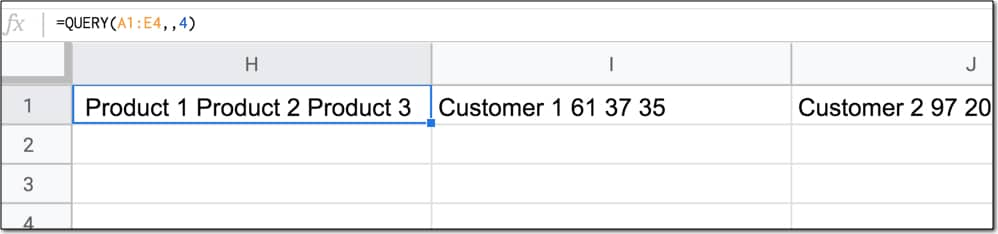Now that is interesting!

And it’s at the heart of how this crazy formula works.

Let’s build it up in steps, following the Onion Method.

The innermost IF function is (note the `value_if_false` argument is empty):

`=ArrayFormula(IF(Sheet1!B2:Z<>"", Sheet1!A2:A&"🐠"&Sheet1!B1:1&"🐠"&Sheet1!B2:Z&"🌶", ))`

which gives the following output: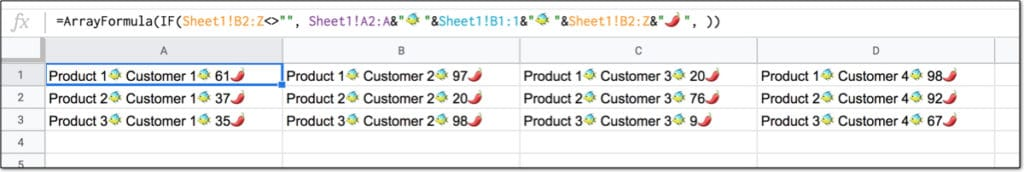For every row of data, the formula joins them such that each cell has a unique combination of product, customer and value.

`=ArrayFormula(QUERY(TRANSPOSE(IF(Sheet1!B2:Z<>"", Sheet1!A2:A&"🐠"&Sheet1!B1:1&"🐠"&Sheet1!B2:Z&"🌶", )),,500000))`

This gives a #REF! error, with the message “Result was not automatically expanded, please insert more columns (699).”

The array output is too wide for our current Sheet.

Wrap it with a transpose function to fix this and get all the data in a single column:

`=ArrayFormula(TRANSPOSE(QUERY(TRANSPOSE(IF(Sheet1!B2:Z<>"", Sheet1!A2:A&"🐠"&Sheet1!B1:1&"🐠"&Sheet1!B2:Z&"🌶", )),,500000)))`

Use a second QUERY function with this headers trick to bring these values together:

`=ArrayFormula(TRANSPOSE(QUERY(TRANSPOSE(QUERY(TRANSPOSE(IF(Sheet1!B2:Z<>"", Sheet1!A2:A&"🐠"&Sheet1!B1:1&"🐠"&Sheet1!B2:Z&"🌶", )),,500000)),,500000)))`

Now we basically just split this up based on the fish “🐠” and chili pepper “🌶” symbols that we used to separate the data packets.

Here’s the first split and transpose:

`=ArrayFormula(TRANSPOSE(SPLIT(TRANSPOSE(QUERY(TRANSPOSE(QUERY(TRANSPOSE(IF(Sheet1!B2:Z<>"", Sheet1!A2:A&"🐠"&Sheet1!B1:1&"🐠"&Sheet1!B2:Z&"🌶", )),,500000)),,500000)),"🌶")))`

By now, our data looks like this, which is getting closer: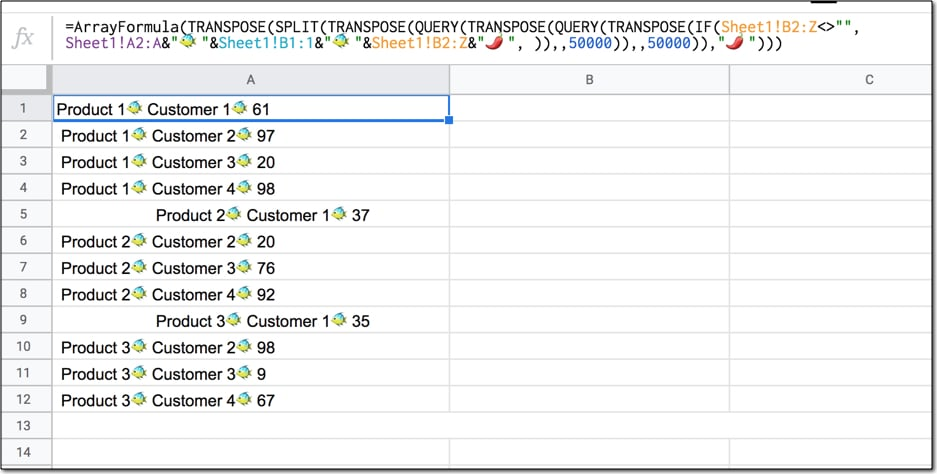Use the TRIM function to fix those unsightly spacing issues.

Next, split it again across the tropical fish:

`=ArrayFormula(SPLIT(TRIM(TRANSPOSE(SPLIT(TRANSPOSE(QUERY(TRANSPOSE(QUERY(TRANSPOSE(IF(Sheet1!B2:Z<>"", Sheet1!A2:A&"🐠"&Sheet1!B1:1&"🐠"&Sheet1!B2:Z&"🌶", )),,500000)),,500000)),"🌶"))),"🐠"))`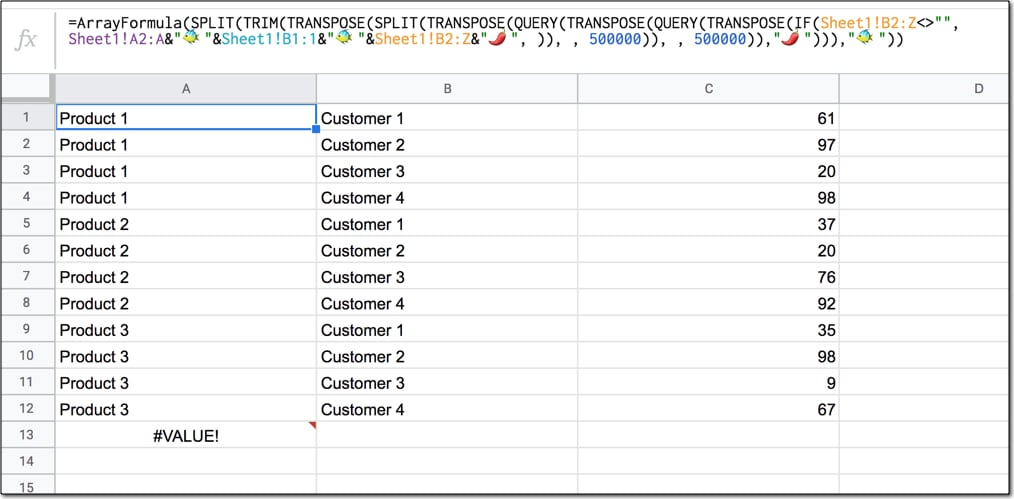Nearly there now!

Remove the #VALUE! error with an IFERROR wrapper function. Use a QUERY wrapper to re-order the rows and columns as required. The OFFSET removes a blank row from showing up in the table. The formula now looks like this:

`=ArrayFormula(QUERY(IFERROR(SPLIT(TRIM(TRANSPOSE(SPLIT(TRANSPOSE(QUERY(TRANSPOSE(QUERY(TRANSPOSE(IF(Sheet1!B2:Z<>"", Sheet1!A2:A&"🐠"&Sheet1!B1:1&"🐠"&Sheet1!B2:Z&"🌶", )),,500000)),,500000)),"🌶"))),"🐠"),""),"SELECT Col2, Col1, Col3 ORDER BY Col2 OFFSET 1"))`

And the output like this:The final step is borrowed from Solution 3 above, namely combining a static header row with array literals.

`=ArrayFormula({"Customer","Product","Value"; MAIN_FORMULA })`

Now we can insert our formula into this construction, in place of the MAIN_FORMULA placeholder:

```=ArrayFormula({"Customer","Product","Value"; QUERY(IFERROR(SPLIT(TRIM(TRANSPOSE(SPLIT(TRANSPOSE(QUERY(TRANSPOSE(QUERY(TRANSPOSE(IF(Sheet1!B2:Z<>"", Sheet1!A2:A&"🐠"&Sheet1!B1:1&"🐠"&Sheet1!B2:Z&"🌶", )), , 500000)), , 500000)),"🌶"))),"🐠"),""),"SELECT Col2, Col1, Col3 ORDER BY Col2 OFFSET 1",0)})```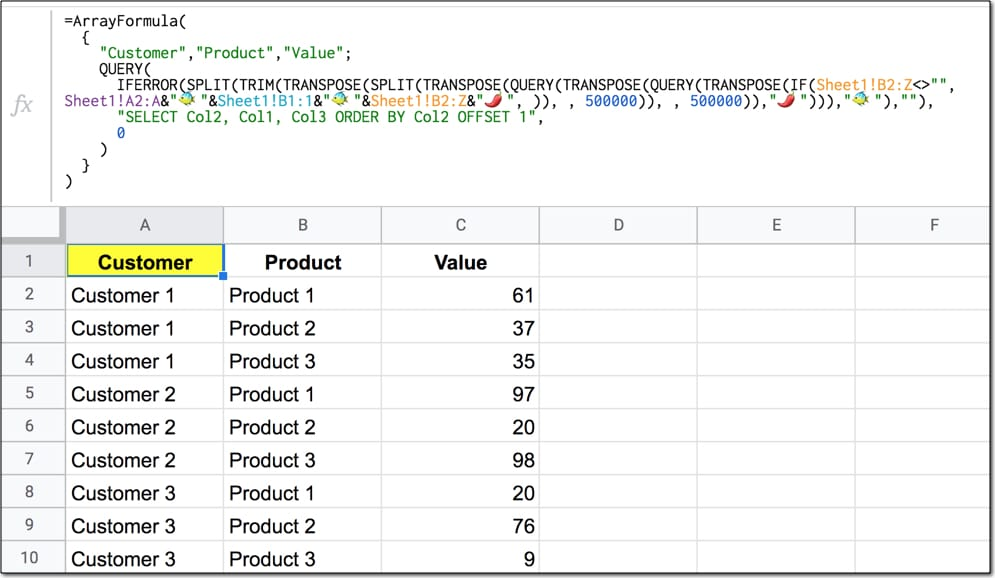## 22 thoughts on “Unpivot In Google Sheets With Formulas, Or How To Turn Wide Data Into Tall Data”

1.Helmanfrow says:

There is a fifth solution.
I was looking for a solution to this very problem several months ago and after digging through forums for a couple of hours I found a custom function creatively named ‘UNPIVOT’ I no longer remember what forum led me to it but I found what appears to be the source:
https://gist.github.com/philippchistyakov/57390ec98dcaea4502fabc5a32242b3a
I use it on a daily basis and it’s delightful.
Enjoy!

1.Victor says:

Hi. This looks quite interesting. I tried to use it on the same example but couldn’t make it work. I get a message indicating “no data”: throw new Error(‘no data’); (line 21).
Is there anything I’m missing? I don’t have much experience with GAS so sorry if this is a silly question. Thank you!

1.Victor says:

Solved it myself! Now I understand. Thank you so much for all this info. Really useful!

1.Sean says:

How did you solve this?

1.Harrison Morrow says:

Right, this is my exact problem… If I find the solutions I will post it.

1.Harrison Morrow says:

So… it’s because I didn’t understand it being a custom function and you have to use it in the sheet where you want it to work. Data being the array you want to use.

The original link for the function is on stack exchange and includes an example.

https://stackoverflow.com/a/43681525

1.Helmanfrow says:

Yes! It was on StackExchange. Good call.

2.Manish says:

Power query of excel does this unpivot in a ziffy with a single click.

2.Markie says:

Helmanfrowsays, that custom formula is awesome!!!

2.S k srivastava says:

This sheet gives single cell formula ( without using script) to unpivot a matrix by using FLATTEN function.
http://tiny.cc/unpivot
Honestly speaking I don’t think it can be further simplified.

1.Ben says:

Wow, very cool, S k srivastava! Thanks for sharing and to Pransanth for finding and documenting the FLATTEN function. I was not aware of that one!

1.S k srivastava says:

Thanx. I modified it a bit. Now it can handle even more than one fixed column.

1.Klaas says:

Is it possible to skip the empty cells? So for example skip Row1, Col2 in the result?

2.Matthew King says:

Prashanth did not find the flatten function. It was found by a user on the Google Sheets Community forums. This link is the discovery on 3/16/20.

3.Ronald Adrianta says:

I modified the formula to Solution 4 a little bit to handle product names with double spaces (for example: Product Name). The original formula would trim those double spaces into single space.

=ArrayFormula(SPLIT(REGEXREPLACE(TRANSPOSE(SPLIT(TRANSPOSE(QUERY(TRANSPOSE(QUERY(TRANSPOSE(IF(Sheet1!B2:Z””, Sheet1!A2:A&”🐠”&Sheet1!B1:1&”🐠”&Sheet1!B2:Z&”🌶”, )),,500000)),,500000)),”🌶”)),”^\s*”,””),”🐠”))

4.Hannah L says:

I added more columns and was having issues if not all columns had data in them. To fix this I modified solution 4 to put a space with each fish when creating the formula, as I found when there was an empty column of data (so you ended up with two fish next to each other before the final split), it would miss a column, but adding a space in (but not as the split identifier) made it work, and the columns all line up. Just need to add an additional trim to get rid of the extra spaces after the final split.

=ArrayFormula(
{
“Product”,”Other”,”one more”,”Customer”,”Value”;
QUERY(
iferror(trim(split(TRIM(TRANSPOSE(SPLIT(TRANSPOSE(QUERY(TRANSPOSE(QUERY(TRANSPOSE(IF(Sheet1!D2:AA””, Sheet1!A2:A&” 🐠”&Sheet1!B2:B&” 🐠”&Sheet1!C2:C&” 🐠”&Sheet1!D1:1&” 🐠”&Sheet1!D2:AA&”🌶”, )), , 500000)), , 500000)),”🌶”))),”🐠”)),””),
“SELECT Col1, Col2, Col3, Col4, Col5”,
0
)
}
)

1.Hannah L says:

Actually, now i have an issue with the above, where the final trim is somehow turning all Numeric and date fields into “text” and gsheets doesn’t seem to want to let me format them as numbers? Any suggestions appreciated.

5.John says:

Hi Ben, thank you for sharing the undocumented FLATTEN() method; it was exactly what I was looking for!

6.Yogy Namara says:

This seems overly complicated to me. You can use & to do most of the work, like this:

=arrayformula(split(flatten(arrayformula(A2:A4 &”🐠”& B1:E1 &”🐠”& B2:E4)),”🐠”))

1.Ben says:

Yes, agreed! But the article was written before I was aware of the flatten function 😉

2.Mark says:

This is great, but I have a question. What if your table has blank values, and in your “unpivoted” table you don’t want those rows to show up? Is there any way to remove them by altering this formula? For example, in the above table, B2 has a blank value (not 0, just blank). In my results, I do not want to see a row for Customer 1 – Product 1 since the Value would be blank.

7.Oscar says:

Great Solution, thanks for sharing.